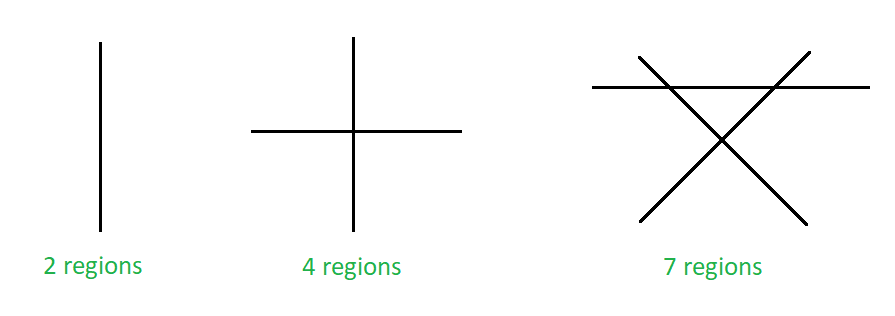# Maximum number of region in which N non-parallel lines can divide a plane

• Difficulty Level : Medium
• Last Updated : 28 Jul, 2022

Given N‘, the number of non-parallel lines. The task is to find the maximum number of regions in which these lines can divide a plane.
Examples:

Input : N = 3
Output : 7
Input : N = 2
Output :Maximum number of regions on a plane formed due to non-parallel lines

Approach: The above image shows the maximum number of regions a line can divide a plane. One line can divide a plane into two regions, two non-parallel lines can divide a plane into 4 regions and three non-parallel lines can divide into 7 regions, and so on. When the nth line is added to a cluster of (n-1) lines then the maximum number of extra regions formed is equal to n.
Now solve the recursion as follows:

L(2) – L(1) = 2 … (i)
L(3) – L(2) = 3 … (ii)
L(4) – L(3) = 4 … (iii)
. . .
. . .
L(n) – L(n-1) = n ; … (n)
Adding all the above equation we get,
L(n) – L(1) = 2 + 3 + 4 + 5 + 6 + 7 + …… + n ;
L(n) = L(1) + 2 + 3 + 4 + 5 + 6 + 7 + …… + n ;
L(n) = 2 + 2 + 3 + 4 + 5 + 6 + 7 + …… + n ;
L(n) = 1 + 2 + 3 + 4 + 5 + 6 + 7 + …… + n + 1 ;
L(n) = n ( n + 1 ) / 2 + 1 ;

The number of region in which N non-parallel lines can divide a plane is equal to N*( N + 1 )/2 + 1.
Below is the implementation of the above approach:

## C++

 `// C++ program to implement the above problem` `#include ``using` `namespace` `std;` `// Function to find the maximum``// number of regions on a plane``void` `maxRegions(``int` `n)``{``    ``int` `num;``    ``num = n * (n + 1) / 2 + 1;` `    ``// print the maximum number of regions``    ``cout << num;``}` `// Driver code``int` `main()``{``    ``int` `n = 10;` `    ``maxRegions(n);` `    ``return` `0;``}`

## Java

 `// Java program to implement the above problem``class` `GFG``{` `    ``// Function to find the maximum``    ``// number of regions on a plane``    ``static` `void` `maxRegions(``int` `n)``    ``{``        ``int` `num;``        ``num = n * (n + ``1``) / ``2` `+ ``1``;` `        ``// print the maximum number of regions``        ``System.out.println(num);;``    ``}` `    ``// Driver code``    ``public` `static` `void` `main(String[] args)``    ``{``        ``int` `n = ``10``;``        ``maxRegions(n);``    ``}``}` `// This code is contributed by 29AjayKumar`

## Python3

 `# Python3 program to implement``# the above problem` `# Function to find the maximum``# number of regions on a plane``def` `maxRegions(n):``    ``num ``=` `n ``*` `(n ``+` `1``) ``/``/` `2` `+` `1` `    ``# print the maximum number``    ``# of regions``    ``print``(num)` `# Driver code``n ``=` `10` `maxRegions(n)` `# This code is contributed``# by Mohit Kumar`

## C#

 `// C# program to implement the above problem``using` `System;``    ` `class` `GFG``{` `    ``// Function to find the maximum``    ``// number of regions on a plane``    ``static` `void` `maxRegions(``int` `n)``    ``{``        ``int` `num;``        ``num = n * (n + 1) / 2 + 1;` `        ``// print the maximum number of regions``        ``Console.WriteLine(num);``    ``}` `    ``// Driver code``    ``public` `static` `void` `Main(String[] args)``    ``{``        ``int` `n = 10;``        ``maxRegions(n);``    ``}``}` `// This code is contributed by 29AjayKumar`

## Javascript

 ``

Output:

`56`

Time Complexity : O(1)

Space Complexity : O(1) since using constant variables

My Personal Notes arrow_drop_up